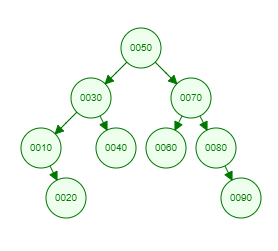Recent Tutorials and Articles
Printing Right View of Binary Tree in Java
Published on: 27th October 2018

This tutorial provides a Java program to print nodes of Binary Tree that will be visible from right view.

# Understanding Right View of a Binary Tree

Right View of a Binary Tree consists of nodes that are visible from Right side of a Binary Tree. This requires level order traversal and printing first node from right side at each level.

Let's consider below Binary Tree -Here is the right view of above Binary tree -

``````50
70
80
90
``````

# Java Implementation of Right View of a Binary Tree

Here is Java code for Binary Tree containing methods for inserting data and printing right view of Binary Tree -

``````package com.aksain.data.structures;

import java.util.Queue;

public class BinaryTreeWithRightView<T extends Comparable<T>> {
private Node root;

private class Node {
T data;
Node left;
Node right;

private Node(T data) {
this.data = data;
}
}

private Node doInsert(T data, Node node) {
if (node == null) {
return new Node(data);
}
int result = data.compareTo(node.data);
if (result < 0) {
node.left = doInsert(data, node.left);
} else if (result > 0) {
node.right = doInsert(data, node.right);
}

return node;
}

public void insert(T data) {
root = doInsert(data, root);
}

private void doPrintRightView(Node root) {
final Queue<Node> queue = new LinkedList<>();

while(!queue.isEmpty()) {
int noOfElementsAtThisLevel = queue.size();
for(int index = 0; index < noOfElementsAtThisLevel; index++) {
Node tmp = queue.poll();
if(index == noOfElementsAtThisLevel - 1) {
System.out.print(tmp.data + " ");
}

if(tmp.left != null) {
}
if(tmp.right != null) {
}
}
System.out.println();
}
}

public void printRightView() {
doPrintRightView(root);
}

public static void main(String[] args) {
final BinaryTreeWithRightView<Integer> binaryTree = new BinaryTreeWithRightView<>();
binaryTree.insert(50);
binaryTree.insert(30);
binaryTree.insert(10);
binaryTree.insert(20);
binaryTree.insert(40);
binaryTree.insert(70);
binaryTree.insert(60);
binaryTree.insert(80);
binaryTree.insert(90);

binaryTree.printRightView();
}

}
``````

Here is the output of above code -

``````50
70
80
90
``````

Thank you for reading through the tutorial. In case of any feedback/questions/concerns, you can communicate same to us through your comments and we shall get back to you as soon as possible.

Published on: 27th October 2018